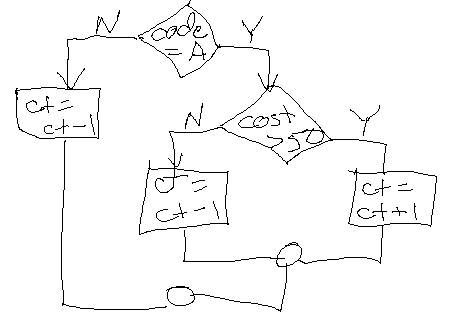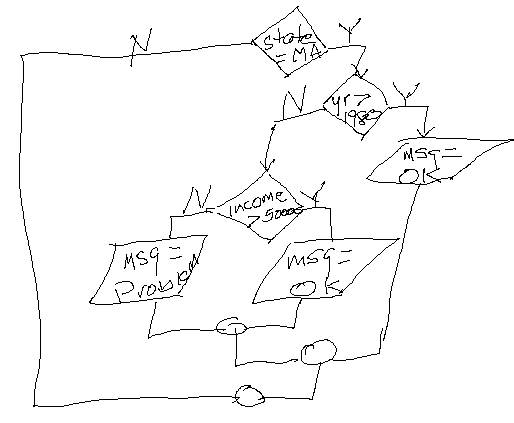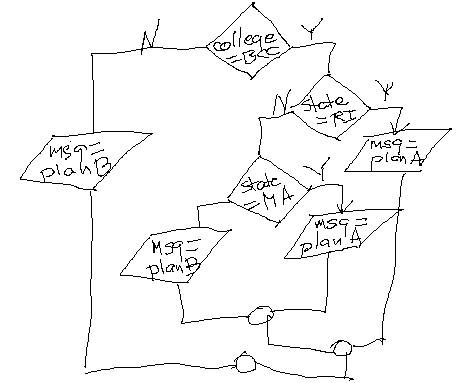Logic Flowchart and Pseudocode Test

This test is going to give you a flowchart for a condition and ask you to answer questions about it.Problem #1:  Assume the following:

·        1 will be put into the memory variable ct

·        A will be put into the memory variable code

·        25 will be put into the memory variable cost

After following the logic in the flowchart, what will be in the memory variable ct?

Problem #2:  Assume the following:

·        the memory variable ct will contain your answer from problem #1

·        A will be put into the memory variable code

·        100 will be put into the memory variable cost

After following the logic in the flowchart, what will be in the memory variable ct?

Problem #3:  Assume the following:

·        the memory variable ct will contain your answer from problem #2

·        B will be put into the memory variable code

·        200 will be put into the memory variable cost

After following the logic in the flowchart, what will be in the memory variable ct?

Problem #4:  Assume the following:

·        the memory variable ct will contain your answer from problem #3

·        A will be put into the memory variable code

·        75 will be put into the memory variable cost

After following the logic in the flowchart, what will be in the memory variable ct?

Problem #5:  Assume the following:

·        the memory variable ct will contain your answer from problem #4

·        A will be put into the memory variable code

·        75 will be put into the memory variable cost

After following the logic in the flowchart, what will be in the memory variable ct?

In the next section, I want you to tell me what pseudocode accurately portrays the logic show in the flowchart.Problem #6: Does this pseudocode accurately portray the flowchart logic? Yes or No

if code = “A”

if cost > 50

ct = ct + 1

else

ct = ct – 1

end if

end if

Problem #7: Does this pseudocode accurately portray the flowchart logic? Yes or No

if code = “A” and cost > 50

ct = ct + 1

else

ct = ct – 1

end if

Problem #8: Does this pseudocode accurately portray the flowchart logic? Yes or No

if code = “A”

if cost > 50

ct = ct + 1

else

ct = ct – 1

end if

else

ct = ct - 1

end if

Now I want to look at another flowchart and process it with the data you are given.Problem #9:  Assume the following:

·        MA will be put into the memory variable state

·        1987 will be put into the memory variable yr

·        25000 will be put into the memory variable income

·        the memory variable msg will be blank

After following the logic in the flowchart, what will be in the memory variable msg?

Problem #10:  Assume the following:

·        RI will be put into the memory variable state

·        1987 will be put into the memory variable yr

·        25000 will be put into the memory variable income

·        the memory variable msg will be blank

After following the logic in the flowchart, what will be in the memory variable msg?

Problem #11:  Assume the following:

·        MA will be put into the memory variable state

·        1975 will be put into the memory variable yr

·        25000 will be put into the memory variable income

·        the memory variable msg will be blank

After following the logic in the flowchart, what will be in the memory variable msg?

Problem #12:  Assume the following:

·        MA will be put into the memory variable state

·        1975 will be put into the memory variable yr

·        75000 will be put into the memory variable income

·        the memory variable msg will be blank

After following the logic in the flowchart, what will be in the memory variable msg?

In the next section, I want you to tell me what pseudocode accurately portrays the logic show in the flowchart.

.Problem #13: Does this pseudocode accurately portray the flowchart logic? Yes or No

if state = “MA”

if yr > 1980

msg = “OK”

else

if income > 50000

msg = “OK”

else

msg = “Problem”

end if

end if

end if

Problem #14: Does this pseudocode accurately portray the flowchart logic? Yes or No

if state = “MA” and yr > 1980 or income > 50000

msg = “OK”

else

msg = “Problem”

end if

Problem #15: Does this pseudocode accurately portray the flowchart logic? Yes or No

if state = “MA” and (yr > 1980 or income > 50000)

msg = “OK”

else

msg = “Problem”

end if

Problem #16: Does this pseudocode accurately portray the flowchart logic? Yes or No

if state = “MA”

if yr > 1980

msg = “OK”

else

if income > 50000

msg = “OK”

else

msg = “Problem”

end if

end if

else

msg = “Problem”

end if

Problem #17: Does this pseudocode accurately portray the flowchart logic? Yes or No

if state = “MA”

if yr > 1980 or income > 50000

msg = “OK”

else

msg = “Problem”

end if

end if

Now I want to look at another flowchart and process it with the data you are given.Problem #18:  Assume the following:

·        BCC will be put into the memory variable college

·        MA will be put into the memory variable state

·        the memory variable msg will be blank

After following the logic in the flowchart, what will be in the memory variable msg?

Problem #19:  Assume the following:

·        CCCC will be put into the memory variable college

·        RI will be put into the memory variable state

·        the memory variable msg will be blank

After following the logic in the flowchart, what will be in the memory variable msg?

Problem #20:  Assume the following:

·        BCC will be put into the memory variable college

·        NY will be put into the memory variable state

·        the memory variable msg will be blank

After following the logic in the flowchart, what will be in the memory variable msg?

In the next section, I want you to tell me what pseudocode accurately portrays the logic show in the flowchart.Problem #21: Does this pseudocode accurately portray the flowchart logic? Yes or No

if college = “BCC”

if state = “RI”

msg = “planA”

else

if state = “MA”

msg = “planA”

else

msg = “planB”

end if

else

msg = “Plan B”

end if

end if

Problem #22: Does this pseudocode accurately portray the flowchart logic? Yes or No

if college = “BCC” and state = “RI” or state = “MA”

msg = “planA”

else

msg = “planB”

end if

Problem #23: Does this pseudocode accurately portray the flowchart logic? Yes or No

if college = “BCC”

if state = “RI”

msg = “planA”

else

if state = “MA”

msg = “planA”

else

msg = “planB”

end if

end if

else

msg = “planB”

end if

Problem #24: Does this pseudocode accurately portray the flowchart logic? Yes or No

if college = “BCC” and (state = “RI” or state = “MA”)

msg = “planA”

else

msg = “planB”

end if

Problem #25: Does this pseudocode accurately portray the flowchart logic? Yes or No

if college = “BCC”

if state = “RI” or state = “MA”

msg = “planA”

else

msg = “planB”

end if

else

msg = “planB”

end if